## Graphing Transformations of Logarithmic Functions

As we mentioned in the beginning of the section, transformations of logarithmic graphs behave similarly to those of other parent functions. We can shift, stretch, compress, and reflect the parent function $y={\mathrm{log}}_{b}\left(x\right)$ without loss of shape.

## Graphing a Horizontal Shift of $f\left(x\right)={\mathrm{log}}_{b}\left(x\right)$

When a constant c is added to the input of the parent function $f\left(x\right)=\text{log}_{b}\left(x\right)$, the result is a horizontal shift c units in the opposite direction of the sign on c. To visualize horizontal shifts, we can observe the general graph of the parent function $f\left(x\right)={\mathrm{log}}_{b}\left(x\right)$ and for > 0 alongside the shift left, $g\left(x\right)={\mathrm{log}}_{b}\left(x+c\right)$, and the shift right, $h\left(x\right)={\mathrm{log}}_{b}\left(x-c\right)$.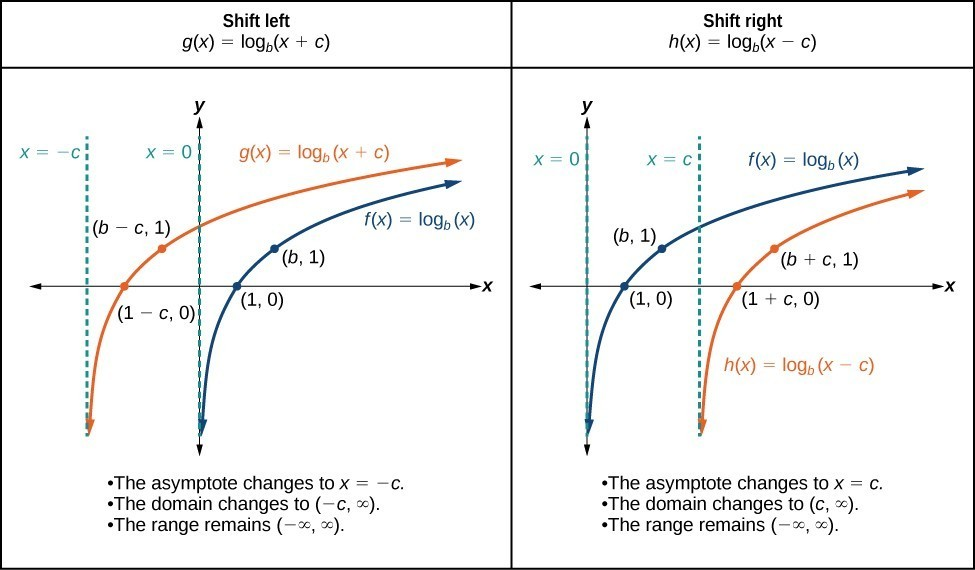Figure 6

### A General Note: Horizontal Shifts of the Parent Function $y=\text{log}_{b}\left(x\right)$

For any constant c, the function $f\left(x\right)={\mathrm{log}}_{b}\left(x+c\right)$

• shifts the parent function $y={\mathrm{log}}_{b}\left(x\right)$ left c units if > 0.
• shifts the parent function $y={\mathrm{log}}_{b}\left(x\right)$ right c units if < 0.
• has the vertical asymptote = –c.
• has domain $\left(-c,\infty \right)$.
• has range $\left(-\infty ,\infty \right)$.

### How To: Given a logarithmic function with the form $f\left(x\right)={\mathrm{log}}_{b}\left(x+c\right)$, graph the translation.

1. Identify the horizontal shift:
1. If c > 0, shift the graph of $f\left(x\right)={\mathrm{log}}_{b}\left(x\right)$ left c units.
2. If < 0, shift the graph of $f\left(x\right)={\mathrm{log}}_{b}\left(x\right)$ right c units.
2. Draw the vertical asymptote = –c.
3. Identify three key points from the parent function. Find new coordinates for the shifted functions by subtracting c from the x coordinate.
4. Label the three points.
5. The Domain is $\left(-c,\infty \right)$, the range is $\left(-\infty ,\infty \right)$, and the vertical asymptote is = –c.

### Example 4: Graphing a Horizontal Shift of the Parent Function $y=\text{log}_{b}\left(x\right)$

Sketch the horizontal shift $f\left(x\right)={\mathrm{log}}_{3}\left(x - 2\right)$ alongside its parent function. Include the key points and asymptotes on the graph. State the domain, range, and asymptote.

### Solution

Since the function is $f\left(x\right)={\mathrm{log}}_{3}\left(x - 2\right)$, we notice $x+\left(-2\right)=x - 2$.

Thus = –2, so < 0. This means we will shift the function $f\left(x\right)={\mathrm{log}}_{3}\left(x\right)$ right 2 units.

The vertical asymptote is $x=-\left(-2\right)$ or = 2.

Consider the three key points from the parent function, $\left(\frac{1}{3},-1\right)$, $\left(1,0\right)$, and $\left(3,1\right)$.

The new coordinates are found by adding 2 to the x coordinates.

Label the points $\left(\frac{7}{3},-1\right)$, $\left(3,0\right)$, and $\left(5,1\right)$.

The domain is $\left(2,\infty \right)$, the range is $\left(-\infty ,\infty \right)$, and the vertical asymptote is = 2.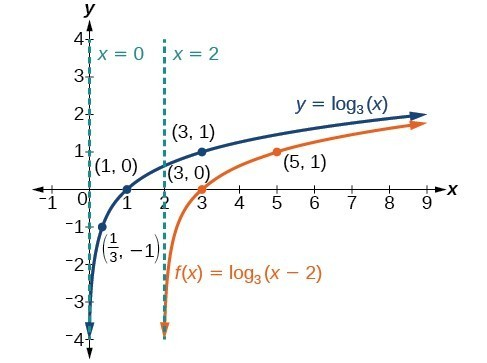Figure 7

### Try It 4

Sketch a graph of $f\left(x\right)={\mathrm{log}}_{3}\left(x+4\right)$ alongside its parent function. Include the key points and asymptotes on the graph. State the domain, range, and asymptote.

Solution

## Graphing a Vertical Shift of $y=\text{log}_{b}\left(x\right)$

When a constant d is added to the parent function $f\left(x\right)={\mathrm{log}}_{b}\left(x\right)$, the result is a vertical shift d units in the direction of the sign on d. To visualize vertical shifts, we can observe the general graph of the parent function $f\left(x\right)={\mathrm{log}}_{b}\left(x\right)$ alongside the shift up, $g\left(x\right)={\mathrm{log}}_{b}\left(x\right)+d$ and the shift down, $h\left(x\right)={\mathrm{log}}_{b}\left(x\right)-d$.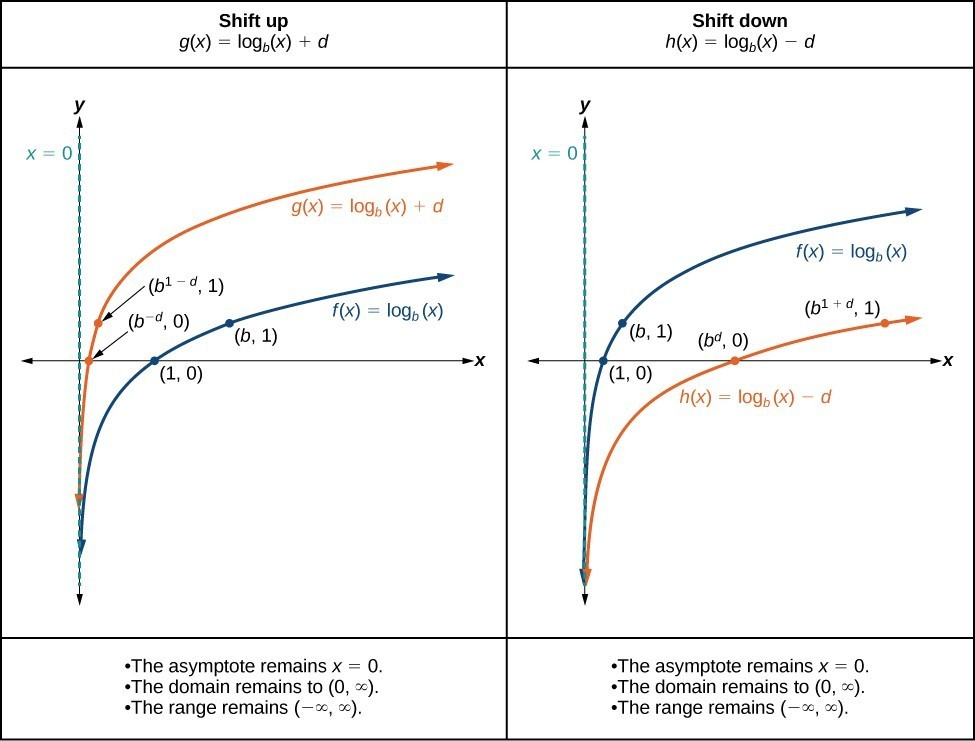Figure 8

### A General Note: Vertical Shifts of the Parent Function $y=\text{log}_{b}\left(x\right)$

For any constant d, the function $f\left(x\right)={\mathrm{log}}_{b}\left(x\right)+d$

• shifts the parent function $y={\mathrm{log}}_{b}\left(x\right)$ up d units if > 0.
• shifts the parent function $y={\mathrm{log}}_{b}\left(x\right)$ down d units if < 0.
• has the vertical asymptote = 0.
• has domain $\left(0,\infty \right)$.
• has range $\left(-\infty ,\infty \right)$.

### How To: Given a logarithmic function with the form $f\left(x\right)={\mathrm{log}}_{b}\left(x\right)+d$, graph the translation.

1. Identify the vertical shift:
1. If > 0, shift the graph of $f\left(x\right)={\mathrm{log}}_{b}\left(x\right)$ up d units.
2. If < 0, shift the graph of $f\left(x\right)={\mathrm{log}}_{b}\left(x\right)$ down units.
2. Draw the vertical asymptote = 0.
3. Identify three key points from the parent function. Find new coordinates for the shifted functions by adding d to the coordinate.
4. Label the three points.
5. The domain is $\left(0,\infty \right)$, the range is $\left(-\infty ,\infty \right)$, and the vertical asymptote is = 0.

### Example 5: Graphing a Vertical Shift of the Parent Function $y=\text{log}_{b}\left(x\right)$

Sketch a graph of $f\left(x\right)={\mathrm{log}}_{3}\left(x\right)-2$ alongside its parent function. Include the key points and asymptote on the graph. State the domain, range, and asymptote.

### Solution

Since the function is $f\left(x\right)={\mathrm{log}}_{3}\left(x\right)-2$, we will notice = –2. Thus < 0.

This means we will shift the function $f\left(x\right)={\mathrm{log}}_{3}\left(x\right)$ down 2 units.

The vertical asymptote is = 0.

Consider the three key points from the parent function, $\left(\frac{1}{3},-1\right)$, $\left(1,0\right)$, and $\left(3,1\right)$.

The new coordinates are found by subtracting 2 from the y coordinates.

Label the points $\left(\frac{1}{3},-3\right)$, $\left(1,-2\right)$, and $\left(3,-1\right)$.

The domain is $\left(0,\infty \right)$, the range is $\left(-\infty ,\infty \right)$, and the vertical asymptote is x = 0.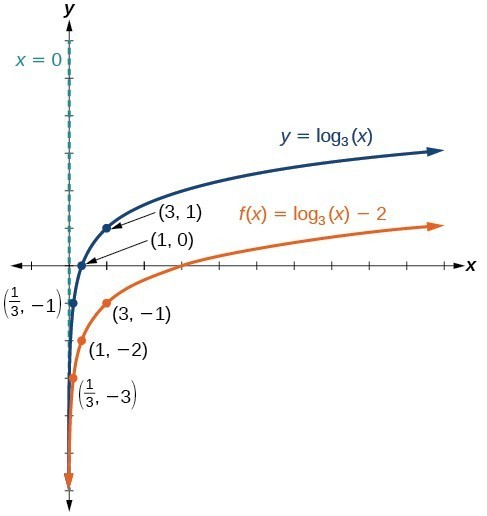Figure 9. The domain is $\left(0,\infty \right)$, the range is $\left(-\infty ,\infty \right)$, and the vertical asymptote is x = 0.

### Try It 5

Sketch a graph of $f\left(x\right)={\mathrm{log}}_{2}\left(x\right)+2$ alongside its parent function. Include the key points and asymptote on the graph. State the domain, range, and asymptote.

Solution

## Graphing Stretches and Compressions of $y=\text{log}_{b}\left(x\right)$

When the parent function $f\left(x\right)={\mathrm{log}}_{b}\left(x\right)$ is multiplied by a constant a > 0, the result is a vertical stretch or compression of the original graph. To visualize stretches and compressions, we set > 1 and observe the general graph of the parent function $f\left(x\right)={\mathrm{log}}_{b}\left(x\right)$ alongside the vertical stretch, $g\left(x\right)=a{\mathrm{log}}_{b}\left(x\right)$ and the vertical compression, $h\left(x\right)=\frac{1}{a}{\mathrm{log}}_{b}\left(x\right)$.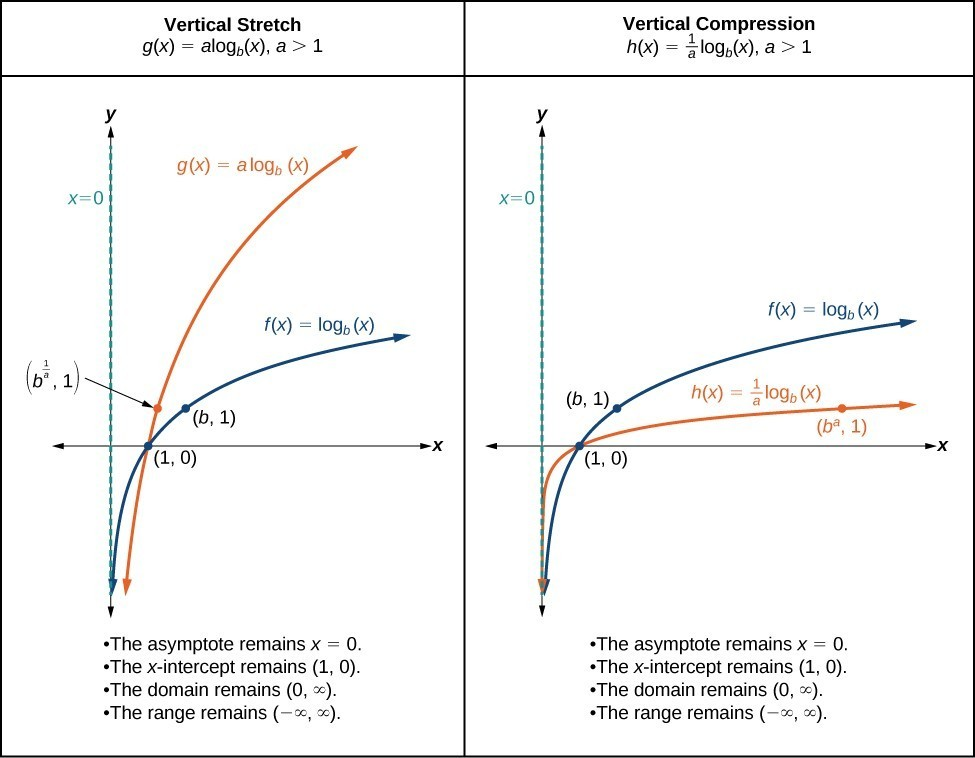Figure 10

### A General Note: Vertical Stretches and Compressions of the Parent Function $y=\text{log}_{b}\left(x\right)$

For any constant a > 1, the function $f\left(x\right)=a{\mathrm{log}}_{b}\left(x\right)$

• stretches the parent function $y={\mathrm{log}}_{b}\left(x\right)$ vertically by a factor of a if > 1.
• compresses the parent function $y={\mathrm{log}}_{b}\left(x\right)$ vertically by a factor of a if 0 < < 1.
• has the vertical asymptote = 0.
• has the x-intercept $\left(1,0\right)$.
• has domain $\left(0,\infty \right)$.
• has range $\left(-\infty ,\infty \right)$.

### How To: Given a logarithmic function with the form $f\left(x\right)=a{\mathrm{log}}_{b}\left(x\right)$, $a>0$, graph the translation.

1. Identify the vertical stretch or compressions:
1. If $|a|>1$, the graph of $f\left(x\right)={\mathrm{log}}_{b}\left(x\right)$ is stretched by a factor of a units.
2. If $|a|<1$, the graph of $f\left(x\right)={\mathrm{log}}_{b}\left(x\right)$ is compressed by a factor of a units.
2. Draw the vertical asymptote = 0.
3. Identify three key points from the parent function. Find new coordinates for the shifted functions by multiplying the y coordinates by a.
4. Label the three points.
5. The domain is $\left(0,\infty \right)$, the range is $\left(-\infty ,\infty \right)$, and the vertical asymptote is x = 0.

### Example 6: Graphing a Stretch or Compression of the Parent Function $y=\text{log}_{b}\left(x\right)$

Sketch a graph of $f\left(x\right)=2{\mathrm{log}}_{4}\left(x\right)$ alongside its parent function. Include the key points and asymptote on the graph. State the domain, range, and asymptote.

### Solution

Since the function is $f\left(x\right)=2{\mathrm{log}}_{4}\left(x\right)$, we will notice = 2.

This means we will stretch the function $f\left(x\right)={\mathrm{log}}_{4}\left(x\right)$ by a factor of 2.

The vertical asymptote is = 0.

Consider the three key points from the parent function, $\left(\frac{1}{4},-1\right)$, $\left(1,0\right)$, and $\left(4,1\right)$.

The new coordinates are found by multiplying the y coordinates by 2.

Label the points $\left(\frac{1}{4},-2\right)$, $\left(1,0\right)$, and $\left(4,\text{2}\right)$.

The domain is $\left(0,\infty \right)$, the range is $\left(-\infty ,\infty \right)$, and the vertical asymptote is = 0.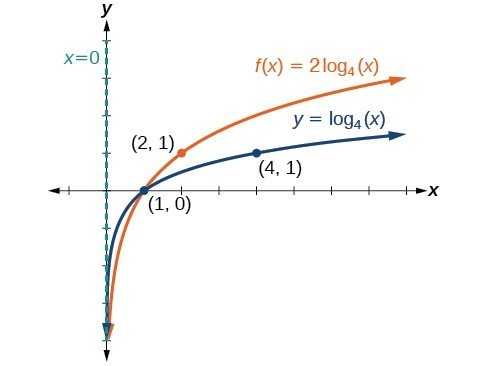Figure 11. The domain is $\left(0,\infty \right)$, the range is $\left(-\infty ,\infty \right)$, and the vertical asymptote is = 0.

### Try It 6

Sketch a graph of $f\left(x\right)=\frac{1}{2}{\mathrm{log}}_{4}\left(x\right)$ alongside its parent function. Include the key points and asymptote on the graph. State the domain, range, and asymptote.

Solution

### Example 7: Combining a Shift and a Stretch

Sketch a graph of $f\left(x\right)=5\mathrm{log}\left(x+2\right)$. State the domain, range, and asymptote.

### Solution

Remember: what happens inside parentheses happens first. First, we move the graph left 2 units, then stretch the function vertically by a factor of 5. The vertical asymptote will be shifted to = –2. The x-intercept will be $\left(-1,0\right)$. The domain will be $\left(-2,\infty \right)$. Two points will help give the shape of the graph: $\left(-1,0\right)$ and $\left(8,5\right)$. We chose = 8 as the x-coordinate of one point to graph because when = 8, + 2 = 10, the base of the common logarithm.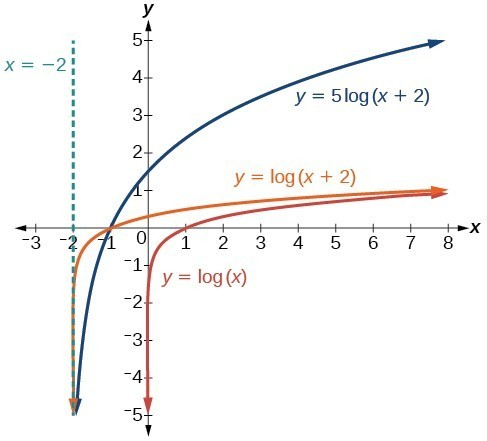Figure 12. The domain is $\left(-2,\infty \right)$, the range is $\left(-\infty ,\infty \right)$, and the vertical asymptote is = –2.

### Try It 7

Sketch a graph of the function $f\left(x\right)=3\mathrm{log}\left(x - 2\right)+1$. State the domain, range, and asymptote.

Solution

## Graphing Reflections of $f\left(x\right)={\mathrm{log}}_{b}\left(x\right)$

When the parent function $f\left(x\right)={\mathrm{log}}_{b}\left(x\right)$ is multiplied by –1, the result is a reflection about the x-axis. When the input is multiplied by –1, the result is a reflection about the y-axis. To visualize reflections, we restrict > 1, and observe the general graph of the parent function $f\left(x\right)={\mathrm{log}}_{b}\left(x\right)$ alongside the reflection about the x-axis, $g\left(x\right)={\mathrm{-log}}_{b}\left(x\right)$ and the reflection about the y-axis, $h\left(x\right)={\mathrm{log}}_{b}\left(-x\right)$.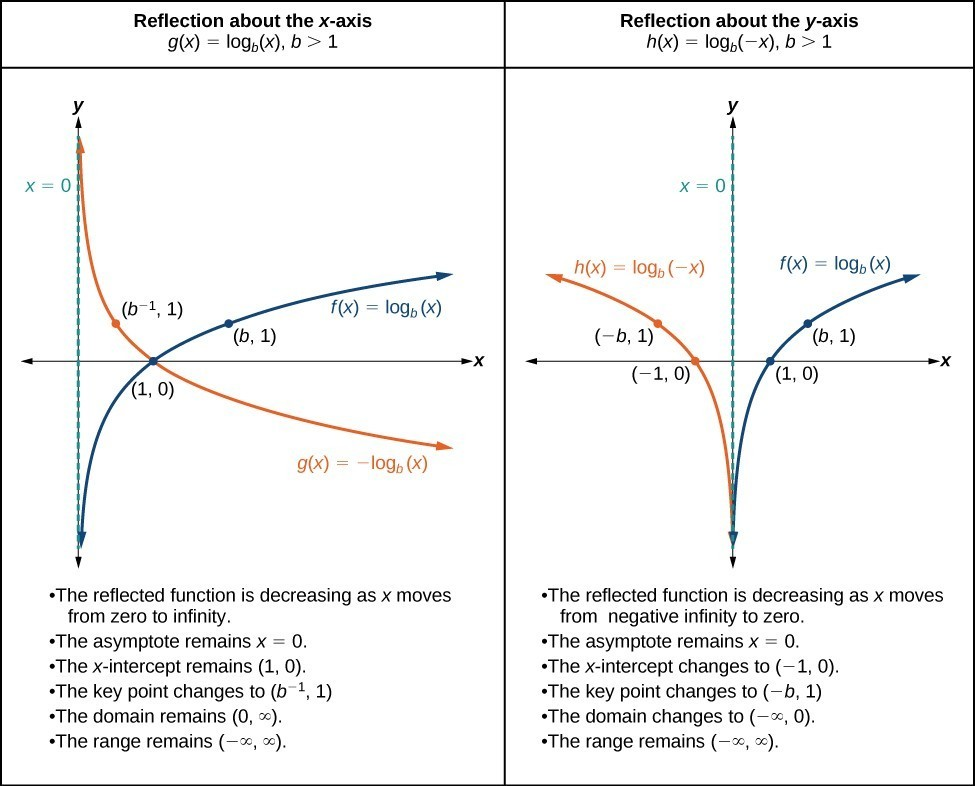Figure 13

### A General Note: Reflections of the Parent Function $y=\text{log}_{b}\left(x\right)$

The function $f\left(x\right)={\mathrm{-log}}_{b}\left(x\right)$

• reflects the parent function $y={\mathrm{log}}_{b}\left(x\right)$ about the x-axis.
• has domain, $\left(0,\infty \right)$, range, $\left(-\infty ,\infty \right)$, and vertical asymptote, = 0, which are unchanged from the parent function.

The function $f\left(x\right)={\mathrm{log}}_{b}\left(-x\right)$

• reflects the parent function $y={\mathrm{log}}_{b}\left(x\right)$ about the y-axis.
• has domain $\left(-\infty ,0\right)$.
• has range, $\left(-\infty ,\infty \right)$, and vertical asymptote, = 0, which are unchanged from the parent function.

### How To: Given a logarithmic function with the parent function $f\left(x\right)={\mathrm{log}}_{b}\left(x\right)$, graph a translation.

$\text{If }f\left(x\right)=-{\mathrm{log}}_{b}\left(x\right)$ $\text{If }f\left(x\right)={\mathrm{log}}_{b}\left(-x\right)$
1. Draw the vertical asymptote, = 0. 1. Draw the vertical asymptote, = 0.
2. Plot the x-intercept, $\left(1,0\right)$. 2. Plot the x-intercept, $\left(1,0\right)$.
3. Reflect the graph of the parent function $f\left(x\right)={\mathrm{log}}_{b}\left(x\right)$ about the x-axis. 3. Reflect the graph of the parent function $f\left(x\right)={\mathrm{log}}_{b}\left(x\right)$ about the y-axis.
4. Draw a smooth curve through the points. 4. Draw a smooth curve through the points.
5. State the domain, $\left(0,\infty \right)$, the range, $\left(-\infty ,\infty \right)$, and the vertical asymptote = 0. 5. State the domain, $\left(-\infty ,0\right)$, the range, $\left(-\infty ,\infty \right)$, and the vertical asymptote = 0.

### Example 8: Graphing a Reflection of a Logarithmic Function

Sketch a graph of $f\left(x\right)=\mathrm{log}\left(-x\right)$ alongside its parent function. Include the key points and asymptote on the graph. State the domain, range, and asymptote.

### Solution

Before graphing $f\left(x\right)=\mathrm{log}\left(-x\right)$, identify the behavior and key points for the graph.

• Since = 10 is greater than one, we know that the parent function is increasing. Since the input value is multiplied by –1, f is a reflection of the parent graph about the y-axis. Thus, $f\left(x\right)=\mathrm{log}\left(-x\right)$ will be decreasing as x moves from negative infinity to zero, and the right tail of the graph will approach the vertical asymptote = 0.
• The x-intercept is $\left(-1,0\right)$.
• We draw and label the asymptote, plot and label the points, and draw a smooth curve through the points.

Figure 14. The domain is $\left(-\infty ,0\right)$, the range is $\left(-\infty ,\infty \right)$, and the vertical asymptote is = 0.

### Try It 8

Graph $f\left(x\right)=-\mathrm{log}\left(-x\right)$. State the domain, range, and asymptote.

Solution

### How To: Given a logarithmic equation, use a graphing calculator to approximate solutions.

1. Press [Y=]. Enter the given logarithm equation or equations as Y1= and, if needed, Y2=.
2. Press [GRAPH] to observe the graphs of the curves and use [WINDOW] to find an appropriate view of the graphs, including their point(s) of intersection.
3. To find the value of x, we compute the point of intersection. Press [2ND] then [CALC]. Select “intersect” and press [ENTER] three times. The point of intersection gives the value of x, for the point(s) of intersection.

### Example 9: Approximating the Solution of a Logarithmic Equation

Solve $4\mathrm{ln}\left(x\right)+1=-2\mathrm{ln}\left(x - 1\right)$ graphically. Round to the nearest thousandth.

### Solution

Press [Y=] and enter $4\mathrm{ln}\left(x\right)+1$ next to Y1=. Then enter $-2\mathrm{ln}\left(x - 1\right)$ next to Y2=. For a window, use the values 0 to 5 for x and –10 to 10 for y. Press [GRAPH]. The graphs should intersect somewhere a little to right of = 1.

For a better approximation, press [2ND] then [CALC]. Select [5: intersect] and press [ENTER] three times. The x-coordinate of the point of intersection is displayed as 1.3385297. (Your answer may be different if you use a different window or use a different value for Guess?) So, to the nearest thousandth, $x\approx 1.339$.

### Try It 9

Solve $5\mathrm{log}\left(x+2\right)=4-\mathrm{log}\left(x\right)$ graphically. Round to the nearest thousandth.

Solution

## Summarizing Translations of the Logarithmic Function

Now that we have worked with each type of translation for the logarithmic function, we can summarize each in the table below to arrive at the general equation for translating exponential functions.

Translations of the Parent Function $y={\mathrm{log}}_{b}\left(x\right)$
Translation Form
Shift

• Horizontally c units to the left
• Vertically d units up
$y={\mathrm{log}}_{b}\left(x+c\right)+d$
Stretch and Compress

• Stretch if $|a|>1$
• Compression if $|a|<1$
$y=a{\mathrm{log}}_{b}\left(x\right)$
Reflect about the x-axis $y=-{\mathrm{log}}_{b}\left(x\right)$
Reflect about the y-axis $y={\mathrm{log}}_{b}\left(-x\right)$
General equation for all translations $y=a{\mathrm{log}}_{b}\left(x+c\right)+d$

### A General Note: Translations of Logarithmic Functions

All translations of the parent logarithmic function, $y={\mathrm{log}}_{b}\left(x\right)$, have the form

$f\left(x\right)=a{\mathrm{log}}_{b}\left(x+c\right)+d$

where the parent function, $y={\mathrm{log}}_{b}\left(x\right),b>1$, is

• shifted vertically up d units.
• shifted horizontally to the left c units.
• stretched vertically by a factor of |a| if |a| > 0.
• compressed vertically by a factor of |a| if 0 < |a| < 1.
• reflected about the x-axis when < 0.

For $f\left(x\right)=\mathrm{log}\left(-x\right)$, the graph of the parent function is reflected about the y-axis.

### Example 10: Finding the Vertical Asymptote of a Logarithm Graph

What is the vertical asymptote of $f\left(x\right)=-2{\mathrm{log}}_{3}\left(x+4\right)+5$?

### Solution

The vertical asymptote is at = –4.

### Analysis of the Solution

The coefficient, the base, and the upward translation do not affect the asymptote. The shift of the curve 4 units to the left shifts the vertical asymptote to = –4.

### Try It 10

What is the vertical asymptote of $f\left(x\right)=3+\mathrm{ln}\left(x - 1\right)$?

Solution

### Example 11: Finding the Equation from a Graph

Find a possible equation for the common logarithmic function graphed in Figure 15.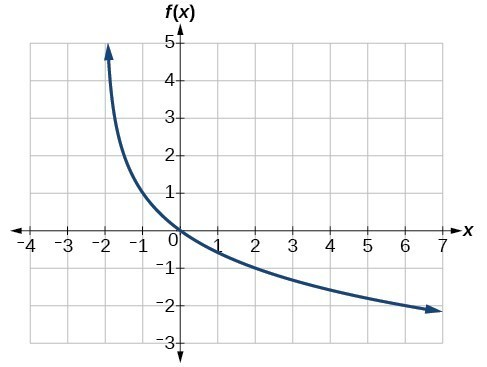Figure 15

### Solution

This graph has a vertical asymptote at = –2 and has been vertically reflected. We do not know yet the vertical shift or the vertical stretch. We know so far that the equation will have form:

$f\left(x\right)=-a\mathrm{log}\left(x+2\right)+k$

It appears the graph passes through the points $\left(-1,1\right)$ and $\left(2,-1\right)$. Substituting $\left(-1,1\right)$,

$\begin{cases}1=-a\mathrm{log}\left(-1+2\right)+k\hfill & \text{Substitute }\left(-1,1\right).\hfill \\ 1=-a\mathrm{log}\left(1\right)+k\hfill & \text{Arithmetic}.\hfill \\ 1=k\hfill & \text{log(1)}=0.\hfill \end{cases}$

Next, substituting in $\left(2,-1\right)$,

$\begin{cases}-1=-a\mathrm{log}\left(2+2\right)+1\hfill & \hfill & \text{Plug in }\left(2,-1\right).\hfill \\ -2=-a\mathrm{log}\left(4\right)\hfill & \hfill & \text{Arithmetic}.\hfill \\ \text{ }a=\frac{2}{\mathrm{log}\left(4\right)}\hfill & \hfill & \text{Solve for }a.\hfill \end{cases}$

This gives us the equation $f\left(x\right)=-\frac{2}{\mathrm{log}\left(4\right)}\mathrm{log}\left(x+2\right)+1$.

### Analysis of the Solution

We can verify this answer by comparing the function values in the table below with the points on the graph in Example 11.

 x −1 0 1 2 3 f(x) 1 0 −0.58496 −1 −1.3219 x 4 5 6 7 8 f(x) −1.5850 −1.8074 −2 −2.1699 −2.3219

### Try It 11

Give the equation of the natural logarithm graphed in Figure 16.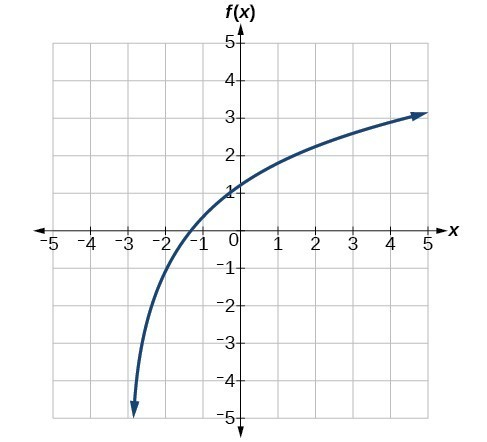Figure 16

Solution

### Q & A

Is it possible to tell the domain and range and describe the end behavior of a function just by looking at the graph?

Yes, if we know the function is a general logarithmic function. For example, look at the graph in Try It 11. The graph approaches x = –3 (or thereabouts) more and more closely, so x = –3 is, or is very close to, the vertical asymptote. It approaches from the right, so the domain is all points to the right, $\left\{x|x>-3\right\}$. The range, as with all general logarithmic functions, is all real numbers. And we can see the end behavior because the graph goes down as it goes left and up as it goes right. The end behavior is that as $x\to -{3}^{+},f\left(x\right)\to -\infty$ and as $x\to \infty ,f\left(x\right)\to \infty$.